# Wave Analyzer

## FFT Spectrum Analyzer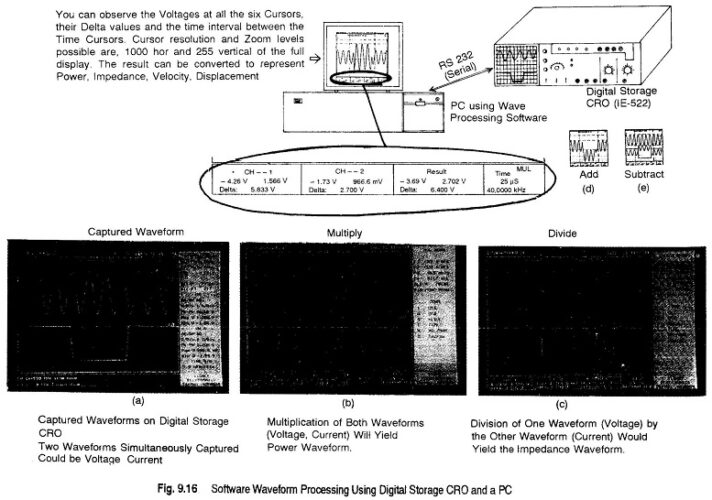FFT Spectrum Analyzer Using a Waveform Processing Software(SS-36): FFT Spectrum Analyzer – The Waveform Processing Software (SS-36) enables one to analyze waveforms very easily. The waveform is captured on a Digital Storage Oscilloscope (DSO) and is transferred to a PC via an RS 232 Serial Interface, which may be in-built in some DSOs. Further, the software …

## Digital Fourier Analyzer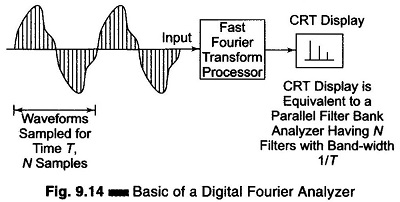Digital Fourier Analyzer: The basic principle of a Digital Fourier Analyzer is shown in Fig. 9.14. The Digital Fourier Analyzer converts the analogue waveform over time period T into N samples. The discrete spectral response Sx (k Δ f); k = 1, 2,…, N which is equivalent to simultaneously obtaining the output from N filters …

## Spectrum Analyzer Block Diagram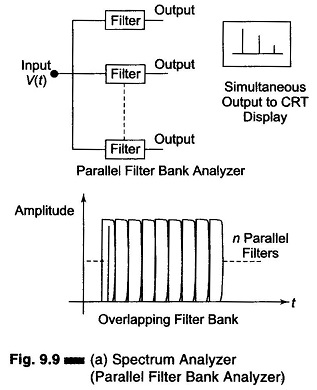Spectrum Analyzer Block Diagram: Spectrum Analyzer Block Diagram – The most common way of observing signals is to display them on an oscilloscope, with time as the X-axis (i.e. amplitude of the signal versus time). This is the time domain. It is also useful to display signals in the frequency domain. The instrument providing this …

## Harmonic Distortion Analyzer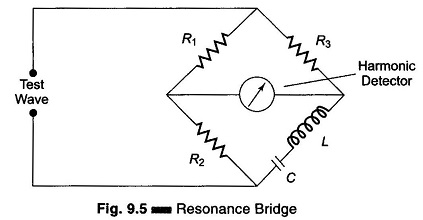Harmonic Distortion Analyzer: A Harmonic Distortion Analyzer measures the total harmonic power present in the test wave rather than the distortion caused by each component. The simplest method is to suppress the fundamental frequency by means of a high pass filter whose cut off frequency is a little above the fundamental frequency. This high pass …

## Heterodyne Wave Analyzer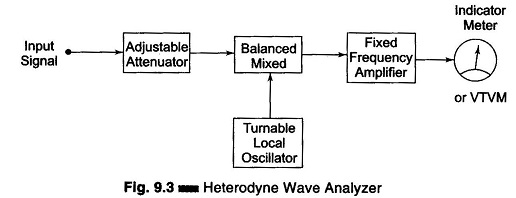Heterodyne Wave Analyzer: Wave analyzers are useful for measurement in the audio frequency range only. For measurements in the RF range and above (MHz range), an ordinary wave analyzer cannot be used. Hence, special types of wave analyzers working on the principle of heterodyning (mixing) are used. These wave analyzers are known as Heterodyne Wave …

## Frequency Selective Wave Analyzer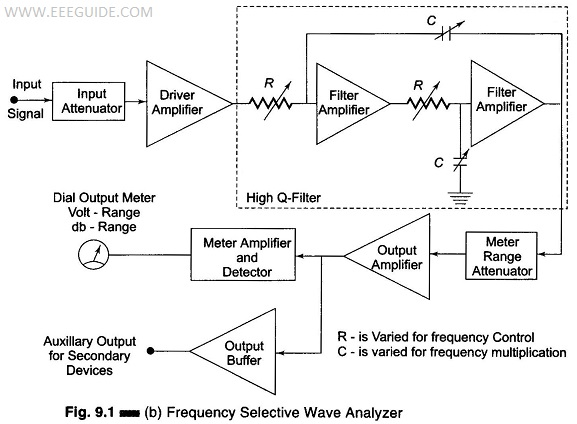Frequency Selective Wave Analyzer: The Frequency Selective Wave Analyzer consists of a very narrow pass-band filter section which can be tuned to a particular frequency within the audible frequency range (20 Hz – 20 kHz). The block diagram of a wave analyzer is as shown in Fig. 9.1(b). The complex wave to be analyzed is …

## What is Wave Analyzer?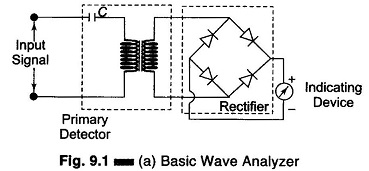What is Wave Analyzer?: It can be shown mathematically that any complex waveform is made up of a fundamental and its harmonics. It is often desired to measure the amplitude of each harmonic or fundamental individually. This can be performed by instruments called wave analyzers. This is the simplest form of analysis in the frequency …

Scroll to Top